Tr

Tr computes the trace of a rectangular matrix.  Some examples are given in
the next two cells.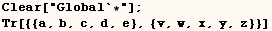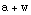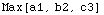Computing the trace of a matrix is a common operation in linear algebra.   Mathematica generalizes the notion of trace to make it so we can compute Tr[vector].   When Tr is given a vector we get the sum of the elements.  The use of (Tr) in  the next cell does the same thing as.  Rob Knapp showed us that when a vector is a packed array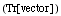is much faster than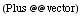.  My timing tests vary, but I found Tr to be at least 25 times faster  when given a packed array.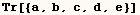Next I give some examples that go down to different levels of a tensor.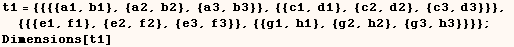By default Tr works at the deepest level, which is level 4 in this example.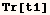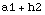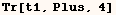Next Tr is used at level 3.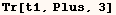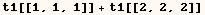Next Tr is used at level 2.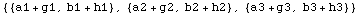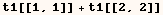Finally Tr is used at level 1.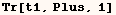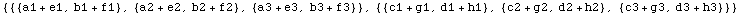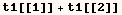Created by Mathematica  (May 16, 2004)

Back to Ted’s Tricks index page